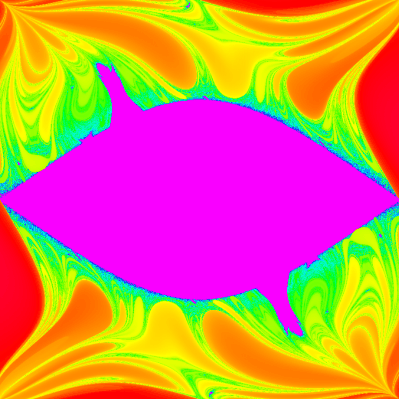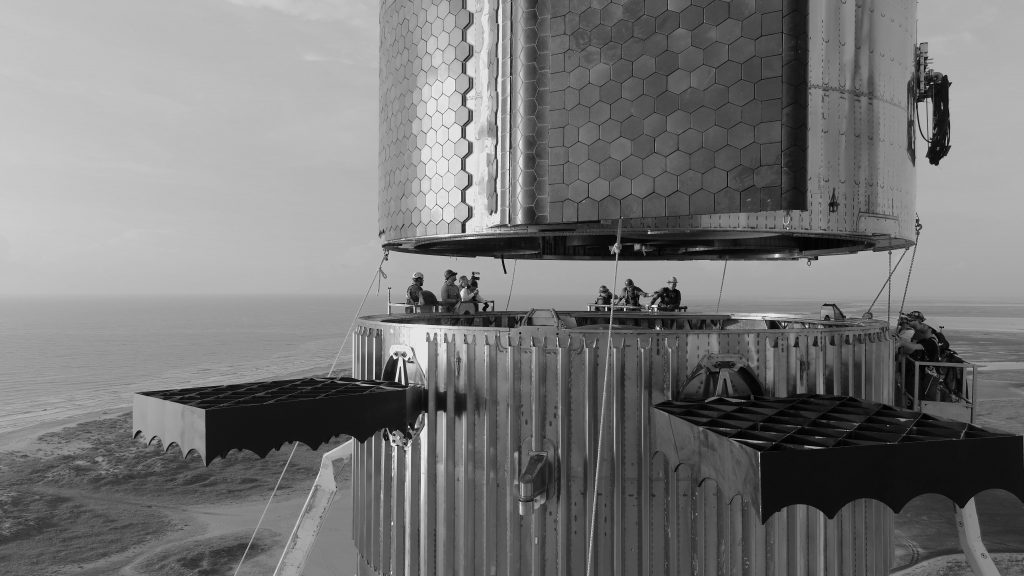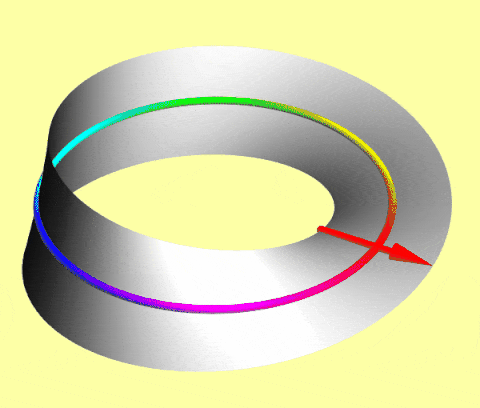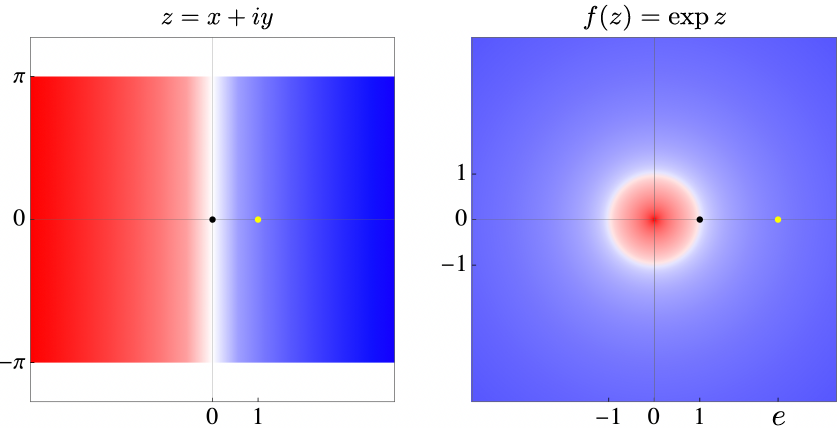## Thinking of Teague

Yesterday, Dr Manz and I went to Lexington, Kentucky to attend the memorial service for Teague Curless.  It was good to gather with Teague’s friends and family so that we could talk about him and remember him, and share our aching hearts with each other.

Teague’s family incorporated a lot of physics into the memorial service, including a beautiful discussion of energy conservation, reminding us that the total energy in the universe has been fixed since its creation, although that energy changes forms. The energy that was Teague is now in different forms, but no less real.  They also read from a lovely commentary by Aaron Freeman about having a physicist speak at your funeral.  These lines really struck me:

You want a physicist to speak at your funeral. You want the physicist to talk to your grieving family about the conservation of energy, so they will understand that your energy has not died…. You want your mother to know that all your energy, every vibration, every BTU of heat, every wave of every particle that was her beloved child remains with her in this world…

[You want the physicist to tell your family]  that all the photons that ever bounced off your face, all the particles whose paths were interrupted by your smile, by the touch of your hair, hundreds of trillions of particles, have raced off like children, their ways forever changed by you.

I know it’s very hard for many of us this Monday, remembering that last Monday, Teague was getting settled on campus and preparing for his senior year.  Things can change so quickly.

For me, the best part of my job is the relationships that I develop with the students. It’s a privilege to get to know you all as you grow and change so much from your first year to your fourth, and to keep in touch as you go out from Wooster and change the world in big ways and small. Thank you for the hugs and calls and emails this week — I am so grateful for all the ways that our community draws together to support one another in tough times like these.

Wooster physics graduates do many things after Wooster, including graduate work. Below is a map of some of the graduate schools they have attended, one dimension of the influence of our department. If you are a recent Wooster physics graduate and don’t see your graduate schools on the map, please contact us, and we will add them.

## For Teague

Sadly and unexpectedly Wooster physics senior Teague Curless ’22 died yesterday. I was fortunate to teach Teague some physics, especially in my Nonlinear Dynamics class last spring. Teague’s semester project beautifully illustrated chaos in a double pendulum — a pendulum swinging from another pendulum, like The Swinging Sticks® kinetic sculpture that silently rotates and librates beside me as I write.

Using Mathematica, Teague numerically integrated the relevant Lagrange equations to simulate the motion of the double pendulum. He then created a two-dimensional initial angles plot of the time for the pendulum to flip as a function of the sub-pendulums’ starting angles, a beautiful high-resolution fractal-like image. I think Teague would have enjoyed the extension below, where I animate the color palette.Rotating hues code time for a double pendulum to flip for different initial angles; central angles are too small to cause flips. Based on Teague Curless’s final Nonlinear Dynamics project.

Posted in Physics, Students, Wooster | 1 Comment

## 21st Century Skyscraper

Recently at its Boca Chica launch site, SpaceX stacked a Starship on a Superheavy booster to briefly form history’s largest rocket, dwarfing the Apollo Saturn V. Both a fit-check and a statement, SpaceX released the photograph below in black & white, which evokes classic mid-20th century skyscraper construction. But this 21st-century skyscraper is designed to fly to Mars — and be fully reusable.

SpaceX has many challenges to overcome to achieve those goals, but watching them try is tremendously exciting. Not since Apollo has space exploration seen such urgency, boldness, and optimism.Stacking the world’s largest rocket evokes classic skyscraper construction (Credit: SpaceX)

Posted in Space Exploration | 1 Comment

## Spinors

Fermions like electrons, protons, and neutrons inhabit a 720° world: 360° rotations negate their quantum states, but 720° rotations restore them.

A simple macroscopic model of such spinors is an arrow translating on a Möbius strip: as the center circle rotates, the attached arrow flips after 360° but flips back after 720°.As the circle rotates, the attached arrow flips after 360° and flips back after 720°

In Dirac notation

$$R_{2\pi}|\psi \rangle = -|\psi \rangle = e^{i \pi}|\psi \rangle,$$

but

$$R_{4\pi}|\psi \rangle = +|\psi \rangle= e^{i 2\pi}|\psi \rangle,$$

where the ket $|\psi\rangle$ is the state, the exponentials are phase factors, and their arguments are phase shifts.

To detect a relative phase shift, send a neutron via two paths, rotate it along one path with a magnetic field (coupled to its magnetic dipole moment), and observe destructive interference for 360° rotations and constructive interference for 720° rotations. (The experiment is harder with charged electrons and protons, whose translation is deflected by the magnetic field.)

## Squares & Cubes

Marvelously, the square of the sum of natural numbers is the sum of their cubes! Equivalently, the sum of their cubes is the square of their sum. This mathematical gem is attributed to Nicomachus of Gerasa who lived almost 2000 years ago.

For example,

$$(1+2+3)^2 = 36 = 1^3 +2^3 + 3^3.$$

More generally,

$$(1 + 2 + \ldots + n)^2 = 1^3 + 2^3 + \cdots n^3$$

or

$$\left( \sum n \right)^2 = \sum n^3.$$

The accompanying animation illustrates the identity, where the cubes can be rearranged into either a square or a sequence of composite cubes of the same total volume.

## Transition

As I transition to emeritus status tomorrow, I reflect on 33 years at Wooster. I am thankful for the freedom I’ve had to design my own courses, including eight first-year seminars; for the flexibility to explore a wide range of research topics, from celestial mechanics to biophysics to dancing in reduced gravity; and for six wonderful yearlong sabbaticals in Atlanta, Portland, Honolulu, and Raleigh. I remember most the many wonderful undergraduates I’ve worked with, including the 73 senior thesis students in the photo mosaic below. Of the 61 peer-reviewed science articles I wrote while at Wooster, 30 of them include 77 undergraduate co-authors (so far).

Posted in Physics, Wooster | 2 Comments

## Archimedes & Euler

A complex function that is its own derivative normalized to one at zero implicitly defines the famous Archimedean and Euler constants of circular motion and exponential growth. Even in a world of strong gravity, where the ratio of a circle’s circumference to its diameter noticeably varied from place to place, this exponential function and the axioms of mathematics would generate these same transcendental numbers.

Assuming the Taylor series expansion

$$f(z) = \sum_{n=0}^\infty \frac{f^{(n)}(0)}{n!} z^n,$$

where $z = x + i y \in \mathbb{C}$ with $x,y \in \mathbb{R}$ and $i = \sqrt{-1}$, the derivative condition

$$f^\prime(z) = f^{(1)}(z) = \sum_{n=1}^\infty \frac{f^{(n)}(0)}{(n-1)!} z^{n-1} = \sum_{n=0}^\infty \frac{f^{(n+1)}(0)}{n!} z^n \equiv f(z) = f^{(0)}(z)$$

coupled with the normalization condition

$$f^{(n+1)}(0) = f^{(n)}(0) = f^{(n-1)}(0) = \cdots = f^{(1)}(0) =f^{(0)}(0) = f(0) \equiv 1$$

implies

$$f(z) = \sum_{n=0}^\infty \frac{z^n}{n!} \equiv \exp z.$$Complex exponential repeatedly maps horizontal strips (left) to the entire complex plane (right), thereby defining the Archimedes and Euler constants. Click for a better view.

Numerically plot this expression to discover two jewels. As in the figure, the exponential function maps the imaginary axis to the unit circle $f(i\mathbb{R}) \rightarrow \mathbb{S}$, with negative real parts forcing complex numbers inside (red) and positive real parts forcing complex numbers outside (blue). The function is exponential on the real axis with e-folding time 1, so $f(1)=e$, but periodic on the imaginary axis with period $\tau$, so $f(z+i\tau)=f(z)$. Specifically, as it maps $1$ to $e$, it maps horizontal strips of height $\tau = 2\pi$ onto the entire complex plane, where

$$\pi =3.14159265358979323846264338327950\ldots$$

and

$$e = 2.71828182845904523536028747135266\ldots$$

are associated with Archimedes and Euler.

## Free-Fall Spinning Tunnels

Jump into an evacuated hole drilled straight through a uniform, static Earth-like sphere. Accelerate to 7.9 km/s (or 18 000 m.p.h.) at the center, then decelerate back to zero at the antipodes 42 minutes later! Step out of the hole upside down — or return 84 minutes after you left.

Last fall, as part of his senior thesis, Yuchen Gan ’21 and I used computer simulations to generalize this famous result to uniform spinning planets, where Coriolis and centrifugal effects force the tunnels into arcs curving away from the center and intersecting the surface in multiple places. We discovered many families of periodic tunnel networks that connect multiple surface locations even at non-equatorial latitudes, as in the animation. Such tunnels could ideally provide energy-free communication and transportation for the planets’ inhabitants.

But in January, in a wonderful aha! moment, we were surprised and delighted by a dramatic perspective change: the motion of an object or passenger (a “terranaut”) freely falling through the tunnel system is both spiky concave arcs with respect to the planet and a smooth convex ellipse with respect to inertial space! We subsequently proved mathematically that the inertial motion is that of a two-dimensional harmonic oscillator, and the ellipses are centered (not focused) on the planet.

Download a higher-resolution QuickTime MOV version of the animation with or without the red elliptic trace.A mass continuously free falls through a tunnel connecting 6 surface points in a spinning planet, simultaneously executing concave spiky motion (with respect to the planet) and convex elliptic motion (with respect to inertial space)